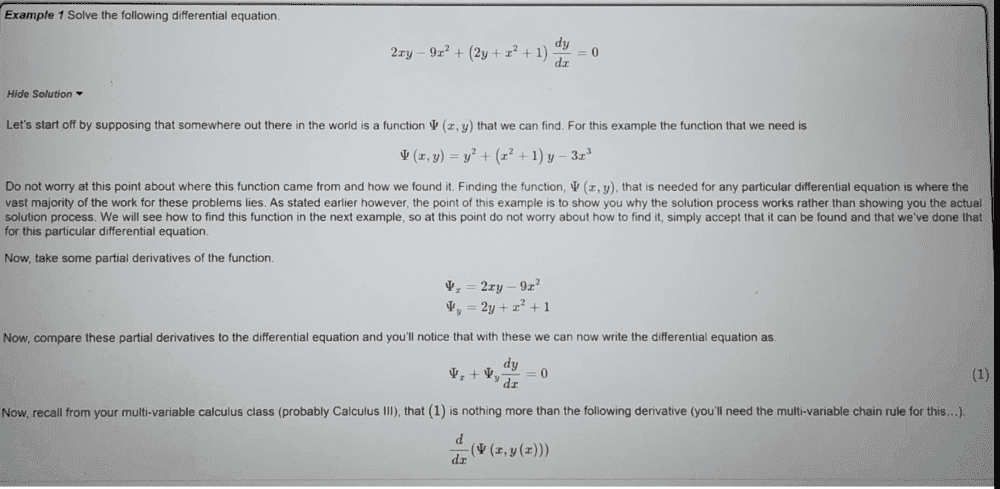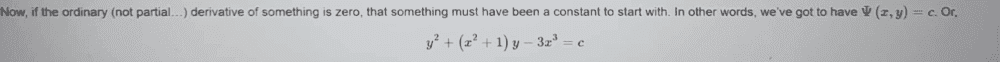# Solve this exact differential equation

Gold Member
Homework Statement:
Solve the exact differential equation
Relevant Equations:
exact equationsnow my approach is different, i just want to check that my understanding on this is correct.

see my working below;

Last edited:
•Delta2

Gold Member
##2xy-9x^2+(2y+x^2+1)\frac {dy}{dx}=0##
##2xy-9x^2dx+(2y+x^2+1)dy=0##
Let ##M(x,y)=2xy-9x^2##
##N(x,y)=2y+x^2+1## Since ##\frac {∂M}{∂y}=2x=\frac {∂N}{∂x}=## then the differential equation is exact.
Therefore, ##\int Mdx## = ##x^2y-3x^3+F(y)##..............................1
and ##\int Ndy## = ##y^2+x^2y+y+c ##................................2
therefore, ##F(y)= y^2+y+c##................................3

therefore, we shall have (from 1 and 3), ## x^2y-3x^3+y^2+y=c##
i understand it this way better, i just want to know if this is also correct.

Last edited:
•Delta2
PeroK
Homework Helper
Gold Member
2020 Award
Looks fine to me.

•chwala
Gold Member
That's how I understand it better from my undergraduate studies...thanks

•Delta2
Mark44
Mentor
i just want to know if this is also correct.
Once you have your solution, it's good practice to check by finding the total derivative of your expression, which you should be able to manipulate back into the form the equation was given in.

Gold Member
The solution is correct, its a textbook question...my interest was on the approach or rather my way of working the problem to realise the solution.
Thanks Mark for your input. Yeah I will use total derivatives to check the solution...

Gold Member
Once you have your solution, it's good practice to check by finding the total derivative of your expression, which you should be able to manipulate back into the form the equation was given in.